PPWIZARD is a free preprocessor for HTML, REXX, Visual Basic or any text files.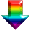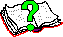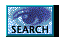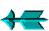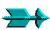\ -> Rexx -> Inbuilt PPWIZARD Functions -> FormatNumber()

 FormatNumber()

This is an inbuilt function function provided by PPWIZARD.

The purpose of this routine is to format a number. Note that the standard rexx function "format" can also do some number formatting.

This routine takes 2 parameters as follows:

1. Number
This is a number, an integer or a number with some decmal places (exampes "10" and "1.234").

2. FormatSpec
This is a string which contains codes which will get replaced by number information (more detail below). If not supplied the then commas are added to the number.

The return code is the formatted value.

 FormatSpec

This is a string which may contain two character long control codes (all of which begin with "%"), if the control code is not recognised then it is ignored. The codes are:

• %%
This is used if you want a percent sign.

• %_
Expands to a single space.

• %,
This indicates that the integer portion of any numbers should have commas added. This would format "1234" as "1,234".

• %N
Replaced with the original number exactly as supplied, for example "%,%N" could be used to add commas.

• %I
Replaced with the integer portion of the original number supplied, for example "%,%I" could be used to add commas.

• %?
This command takes 2 text parameters and looks at the last "number" and will decide which of the 2 text strings to use. Only if the number is exactly one the first string is used. For example "%I %?byte,bytes" could be used. This code will not work in all possible situations (but it will be consistant).

• %K
Return the original number divided by 1024 and set remainder.

• %k
Return the original number divided by 1000 and set remainder.

• %M
Return the original number divided by 1024*1024 and set remainder.

• %m
Return the original number divided by 1000*1000 and set remainder.

• %1
Will output the first decimal place.

• %2
Will output the first 2 decimal places.

• %3
Will output the first 3 decimal places.

• %4
Will output the first 4 decimal places.

• %R
The remainder.

 Example

```#DefineRexx ''
N = 293842944

;--- Display number in MEG (to 4 decimal places) ---
call say 'In MEG: ' || FormatNumber(N, "%,%M.%4")

;--- Display number with commas and byte/bytes ---------------------------
call say 'Number: ' || FormatNumber(N, "%,%N %?byte,bytes;")
call say '1     : ' || FormatNumber(1, "%,%N %?byte,bytes;")
#DefineRexx
```

Saturday May 28 2022 at 2:55pm